# Plot a bar chart of classifications

This is an example showing how to produce a bar chart showing the percentage abundance of each classification in a 2D or 3D array of classifications.

First we shall create a random 3D grid of classifications that can be plotted. Usually you would use a method such as `mcalf.models.ModelBase.classify_spectra()` to classify an array of spectra.

```from mcalf.tests.helpers import class_map as c

t = 3  # Three images
x = 50  # 50 coordinates along x-axis
y = 20  # 20 coordinates along y-axis
n = 5  # Possible classifications [0, 1, 2, 3, 4]

class_map = c(t, x, y, n)  # 3D array of classifications (t, y, x)
```

Next, we shall import `mcalf.visualisation.bar()`.

```from mcalf.visualisation import bar
```

We can now simply plot the 3D array. By default, the first dimension of a 3D array will be averaged to produce a time average, selecting the most common classification at each (x, y) coordinate. This means the percentage abundances will correspond to the most common classification at each coordinate.

```bar(class_map)
```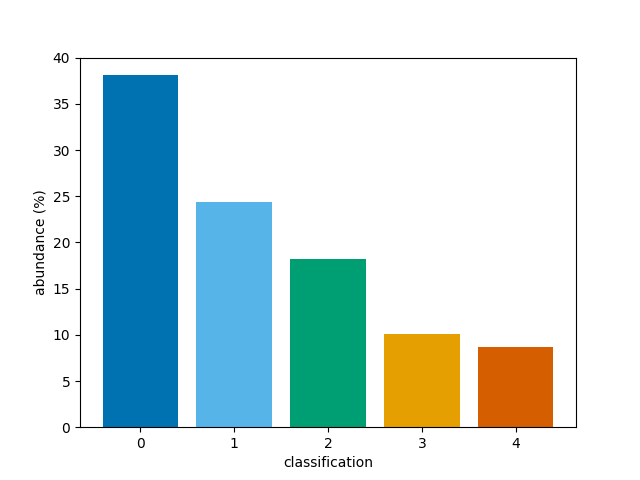```<BarContainer object of 5 artists>
```

Instead, the percentage abundances can be determined for the whole 3D array of classifications by setting `reduce=True`. This skips the averaging process.

```bar(class_map, reduce=False)
```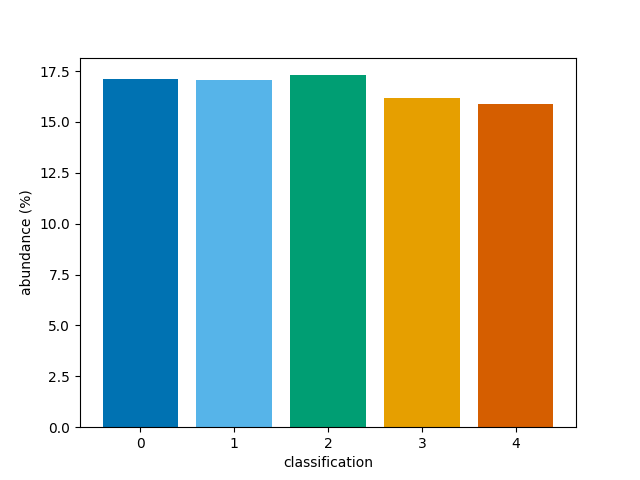```<BarContainer object of 5 artists>
```

Alternatively, a 2D array can be passed to the function. If a 2D array is passed, no averaging is needed, and the `reduce` parameter is ignored.

A narrower range of classifications to be plotted can be requested with the `vmin` and `vmax` parameters. To show bars for only classifcations 1, 2, and 3,

```bar(class_map, vmin=1, vmax=3)
```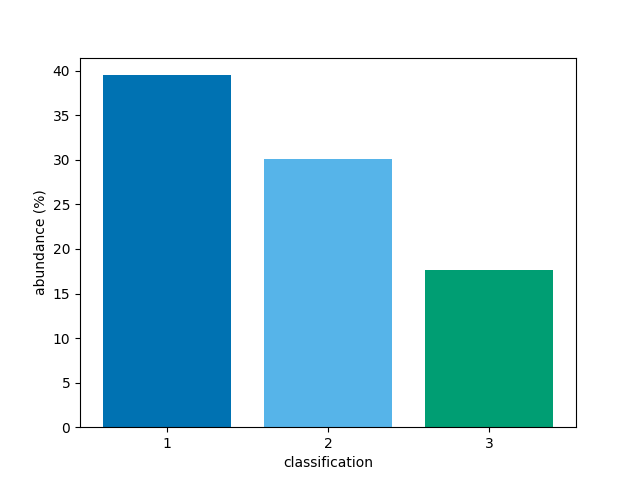```<BarContainer object of 3 artists>
```

An alternative set of colours can be requested. Passing a name of a matplotlib colormap to the `style` parameter will produce a corresponding list of colours for each of the bars. For advanced use, explore the `cmap` parameter.

```bar(class_map, style='viridis')
```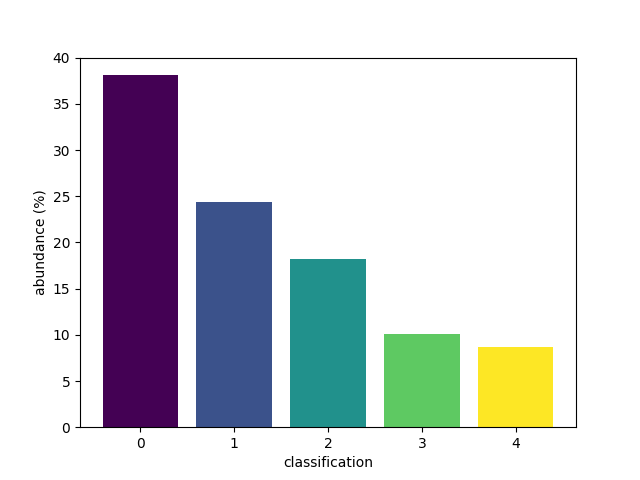```<BarContainer object of 5 artists>
```

The bar function integrates well with matplotlib, allowing extensive flexibility.

```import matplotlib.pyplot as plt

fig, ax = plt.subplots(1, 2, constrained_layout=True)

bar(class_map, vmax=2, style='viridis', ax=ax)
bar(class_map, vmin=3, style='cividis', ax=ax)

ax.set_title('first 3')
ax.set_title('last 2')

ax.set_ylabel('')

plt.show()
```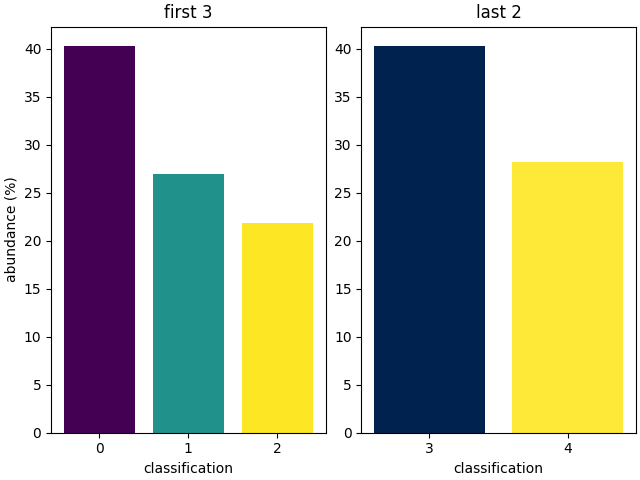Note that `vmin` and `vmax` are applied before the `reduce` value is applied. So setting these ranges can change the calculated abundances for other classifications if `class_map` is 3D and `reduce=True`.

The bars do not add up to 100% as a bar for negative, invalid classifications (and therefore classifications out of the `vmin` and `vmax` range) is not shown.

Total running time of the script: ( 0 minutes 1.081 seconds)

Gallery generated by Sphinx-Gallery